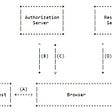# USING C++ PROGRAM ENTER BASIC SALARY AND CALCULATE ITS GROSS SALARY!!!

|BASIC SALARY||GROSS SALARY|

In this program, we going to enter the Basic Salary and calculate its Gross Salary according to the following and display its result.

Basic Salary<25000; HRA=20%; DA=80%.

Basic Salary≥25000; HRA=25%; DA=90%

Basic Salary≥40000; HRA=30%; DA=95%.

→HRA-House Rent Allowance.

→DA-Dearness Allowance.

*Let’s start the program with a header file

“#include<iostream>” →Input-output continuous bytes of data.

“using namespace std;” →used to store variable name.

“float basic,gross,da,hra;

cout<<” Enter basic salary of an employee: ”; “ →output statement.

• Using basic salary we going to calculate hra, da, and gross.

“cin>>basic;” →input statement.

cout →character output

cin →character input

• Now, we are going to use a condition statement(“if statement”)

If statements C++:

C++ has the following conditional statements: Use if to specify a block of code to be executed, if a specified condition is true. Use else to specify a block of code to be executed if the same condition is false. Use else if to specify a new condition to test, if the first condition is false.

## 1 st condition:

“if(basic<25000)

{da=basic*80/100;

hra=basic*20/100;}”

• In “if statement” basic is given as less than 25000, so as given in the question we have taken da=80%(da=basic*80/100;) and hra=20%(hra=basic*20/100;)

If-else statement :

C++ has the following conditional statements: Use if to specify a block of code to be executed, if a specified condition is true. Use else to specify a block of code to be executed if the same condition is false. Use else if to specify a new condition to test, if the first condition is false.

## 2nd condition:

“if(basic≥25000 && basic<40000)

{da=basic*90/100;

hra=basic*25/100;}”

&& →two condition should be satified.

• In this condition (“else if”) basic is given as greater than or equal to 25000 and basic is less than 40000, so that means we have to take da=90%(da=basic*90/100;) and hra=25%(hra=basic*25/100;).

## 3rd condition:

“ else if (basic≥40000)

{da=basic*95/100;

hra=basic*30/100;}”

• In this condition (“else if”) basic is given as greater than equal to 40000, so that means we have to take da=95%(“da=basic*95/100;”) and hra=30%(“hra=basic*30/100;”).

“gross=basic +hra+da;”

• Now we can calculate gross, so we are adding all the three(basic,hra,da)

*basic →user given value.

*hra, da →we have already calculated above.

• Then, we have to print Basic pay, Dearness Allowance, House Rent Allowance, Gross Salary.

*\n →newline character.

*\t →horizontal tab.

*endl →is used to avoid buffer statements and display them in a newline.

--

--

--

## More from Gomathi Keerthana.R.S.

Love podcasts or audiobooks? Learn on the go with our new app.

## Variables## Requirements engineering methods and techniques## Creating User Session for Kafka Consumer using Custom Annotations## Biswap Key Metrics — Starlight Stats!## Gatling, a load testing tool with a difference.## Create a docker image of an offline open street map server## KingSpeed INO event at Babylons, Liquidifty & Tofu NFT launchpads## Definitions of Form## Core memory making: Food Edition## LIVE: Candy Ambulance and Chestnut Grove @ Park Theater, Glens Falls, 02/10/2022## Angular/React — Public client Single Page Applications — a secure practice on where to store the…